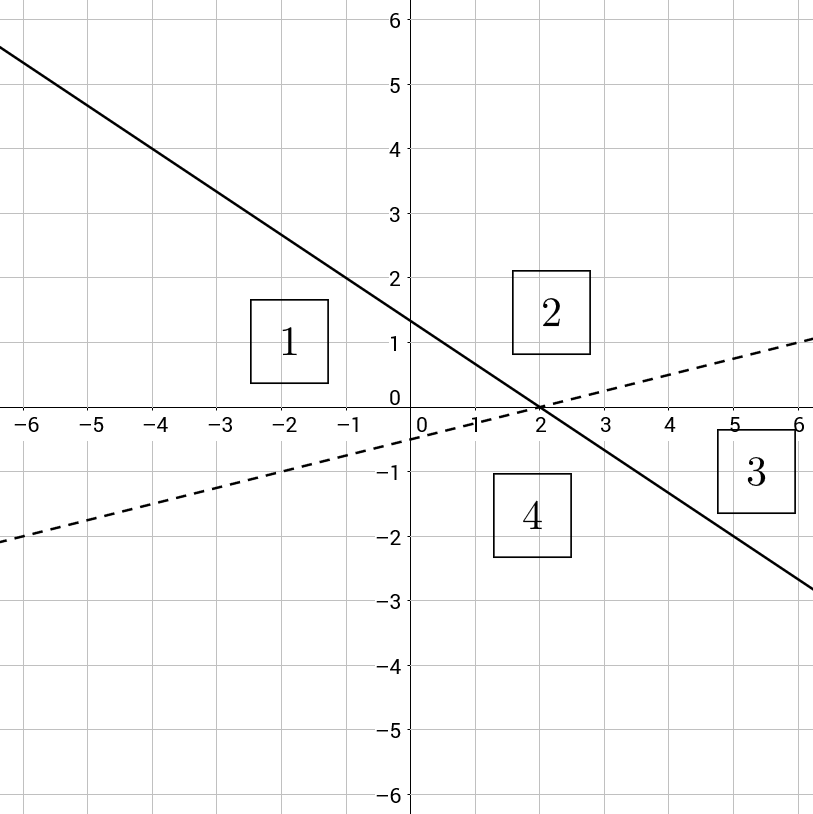Mostly inequality question are very important portion in competitive exams such as clerk, SSC, CGL, placement aptitude, IBPS PO, SBI PO, NICL AO, LIC AAO, SBI associate  clerk, SBI associates PO, CAT and so on. Coded inequality problems are the advanced version of mathematical inequality questions which are frequently asking in many competitive exams. Person who wants to be winning in competitive exams should know this inequality questions. It is also a common topic for all competitive exams. Mostly reasoning part usually carries 5-6 questions from this topic. Candidates have to solve this inequality questions in less than a minutes.

Inequality sign

• >It indicates greater than, neither smaller nor equal
• < It indicates smaller than, less than, neither greater nor equal
• = It indicates equal to, neither greater nor smaller
• <_ It indicates either smaller or equal to, not greater than
• >_ It indicates either greater or equal to not smaller thanHow to solve question on coded inequalities?

Case 1: Equal to

The first step in every equation is to ignore equal. Yes ignore.

Example 1: A=B<C=D

Therefore, A<D

Example 2: A<B=C

Therefore, A<C

Case 2: Same sign

When same sign repeats itself again and again, then that sign would be the answer.

Example 3: A<B<C<D

Therefore, A<D

Example 4: A=B>_C = D>_E

Therefore, A>_E

Case 3: Common sign

(= is never considered as a common sign). So, the every first thing is to ignore (=) as ignore told in case 1.

Example 5: A<B<_C

Therefore, A<C

Example 6:A>_B>C=D>_E>F

Therefore, A>F

Case 4: Opposite sign

Whenever there are opposite sign there are 3 possibilities.

For instance, A>B<_C(No relation), there will be 3 possibilities:

A=C

A>C

A<C

If sign <_ then possibilities are <,=

If sign >_ then possibilities are>_,=

If sign = then possibilities <,>,=

Example 7: A<B<_C=D<_E

Conclusion 1: A<E

Conclusion 2:A<_E

Conclusion 3:A>D

Conclusion 4:B=E

In this question, only conclusion 1 follows.

Conclusion 2 is wrong because common sign between A and E is <

Conclusion 3 is wrong because A< D

Conclusion 4 is wrong because B<_ E (B=E is just a possibility)

How to solve possibilities?

There can be 2 cases:

1. Either – or

If two possibilities are given in the conclusions and both such conclusions have the same variable, We apply ‘either or’ between the conclusions. This means at a time one of the two conclusions will surely be true.

This is the most tricky type of answer. Let’s have a look at the following example in order to understand this point:

Example 8: A<B<_C>D=E<_F

Conclusion 1: A<_D

Conclusion 2: A>D

Conclusion 3: A=D

Conclusion 4: A<_C

In this case correct answer will be Either 1 or 2 follows because there are opposite signs between A and D(<,<_,>) so, in this case there will be 3 possibilities as explained earlier.

Since all three possibilities are present in conclusion 1 or 2, we apply either or case.

Let us now consider another example:

Example 9: A<B>_C=E=F>D

Option 1: A< C

Option 2: A>C

Option 3: F>_B

Option 4: D<F

In this correct answer will be option 4.

In option 1 & option 2,We cannot apply either or case because only 2 possibilities are given but according to the questions, there can be 3 possibilities between A and C.

1. Wrong
2. If all the possible possibilities are not given the conclusion i.e. only some of the possibilities are given, then those conclusion will be considered wrong

Solved examples of coded inequality for reasoning section:

Now , we solve one miscellaneous question to thoroughly understand the concept:

Example 10:

X @ Y means X is not smaller than Y

X % Y means X is not greater than Y

X \$ Y means X is neither smaller than nor greater than Y

X # Y means X is neither greater than nor equal to Y

X * Y means is neither smaller than nor equal to Y

Statements: W % E, P % E, P @ S.

Conclusions:

S * E

W \$ S

1. Only conclusion I follows
2. Only conclusion II follow
3. Both conclusion I and conclusion II follow
4. Neither conclusion I nor conclusion II follows
5. Either conclusion I or conclusion II follows

W % E implies W < E

P % E implies P < E

P @ S implies P> S

Hence, the consolidated relationship is E> P> S and E> W

Conclusion I: S * E implies S > E, which is definitely false.

Conclusion II: W \$ S implies W = S, which may or may not be true as the relationship between S and W cannot be established.

Hence, neither conclusion follows.

Hence, option 4.